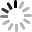Show / Hide
##### Data preview
Source Target Value
Group Size Nodes
##### Graph Information
Basic statistics · Calculate Note

N and E are the number of nodes and links. 〈k〉 and 〈d〉 are the average degree and the average distance, respectively. C and r are the average clustering coefficient and the assortative coefficient. H is the degree heterogeneity. βc is the epidemic threshold of the SIR model.

Degree Histogram · Plot

Communities:

Modularity (Q):

Runtime (s):